## Sheila is flying a kite with a string that makes a 59-degree angle with the sand. If the height of the kite above the ground is 97 fee

Question

Sheila is flying a kite with a string that makes a 59-degree angle with
the sand. If the height of the kite above the ground is 97 feet, how
long is the string? (Please use the exact ratio to calculate the missing

in progress 0
2 weeks 2021-08-28T17:58:54+00:00 1 Answers 0 views 0

113.16 feet

Step-by-step explanation:

Representing the given details appropriately with a right angle triangle. Let the length of the string for the kite be represented by x. Applying required trigonometric function, we have:

Sin θ =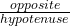Sin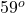=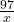x =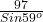=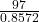= 113.1591

x = 113.16 feet

Thus, the string for the kite is 113.16 feet long.